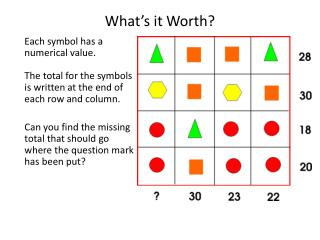DownloadDownload PresentationWhat’s it Worth?

# What’s it Worth?

Download Presentation## What’s it Worth?

- - - - - - - - - - - - - - - - - - - - - - - - - - - E N D - - - - - - - - - - - - - - - - - - - - - - - - - - -
##### Presentation Transcript

1. What’s it Worth? Each symbol has a numerical value. The total for the symbols is written at the end of each row and column. Can you find the missing total that should go where the question mark has been put?

2. In each group each of the letters A through H has one of the eight values listed. No two letters have the same value. The simple arithmetic problems are clues for determining the values of each letter.The three groups are independent. Group 12,5,6,8,10,13,15,21H+D=GE-C=DH+G=AF+H=CE-F=GB+E=A Group 23,4,5,9,12,13,17,22C-A=BH+D=AD+F=GF-A=EC-D=G Group 34,5,6,10,11,14,15,20B-G=HA+E=CF-C=HD-B=AA+H=GE+F=D

3. In addition shown below, each of the letters A, B, C, D, and E represents one of the ciphers from 1 up to 5 (equal letters represent equal ciphers and different letters represent different ciphers). • The first and last ciphers of the sum are given. • A B C D ED A B E CE A A B CA C D A E------------ +9 C B A 0

4. Every alphabet represents a different digit from 1 to 9 to make the calculation. What are they?

5. PQ RST UV W X1. Each letter stands for one of the numbers 1 - 9.2. S + Q = V and S is smaller than Q.3. P = R + U.4. In one of the diagonals, all 3 numbers are perfect squares.5. In one of the two diagonals, (P, T, X or R, T, V) the 3 numbers are in ascending order when read from top to bottom, and each is evenly divisible by the same number, a number larger than 1.WHAT ARE THE NUMBERS? • WHAT ARE THE NUMBERS?9 5 23 4 78 6 1

6. The coloured shapes stand for eleven of the numbers from 0 to 12. Each shape is a different number.Can you work out what they are from the multiplications below? http://nrich.maths.org/5714&part=